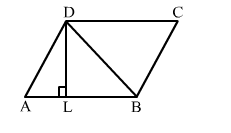# In the given figure, ABCD is a || gm in which DL ⊥ AB. If AB = 10 cm and DL = 4 cm, then the ar(||gm ABCD) = ?

Question:

In the given figure, ABCD is a || gm in which DL ⊥ AB. If AB = 10 cm and DL = 4 cm, then the ar(||gm ABCD) = ?

(a) $40 \mathrm{~cm}^{2}$

(b) $80 \mathrm{~cm}^{2}$

(c) $20 \mathrm{~cm}^{2}$

(d) $196 \mathrm{~cm}^{2}$Solution:

(a) $40 \mathrm{~cm}^{2}$

$\operatorname{ar}(\| g m A B C D)=$ base $\times$ height $=10 \times 4=40 \mathrm{~cm}^{2}$